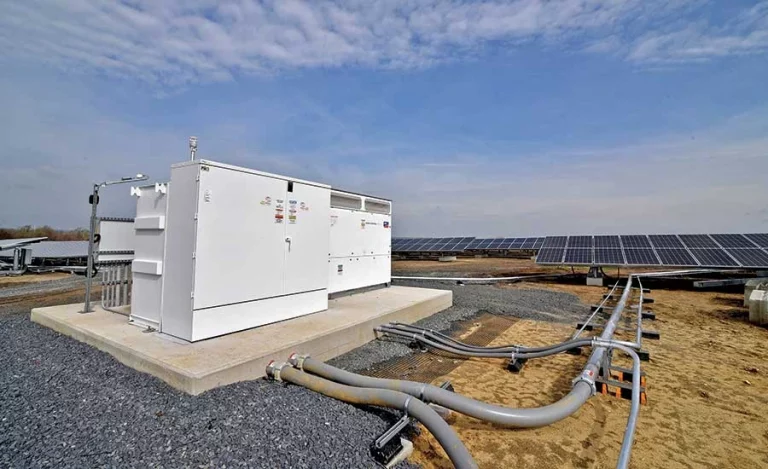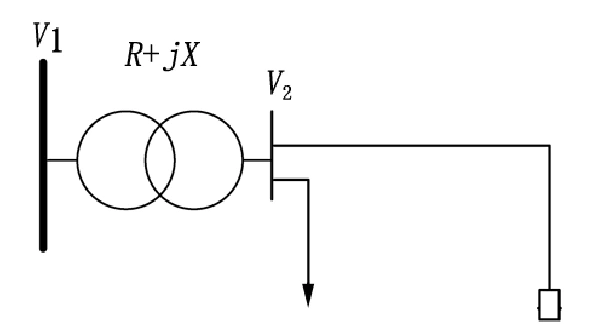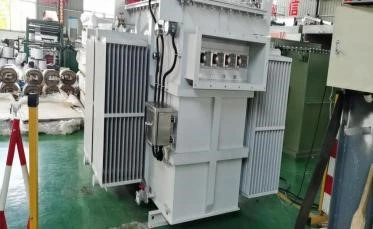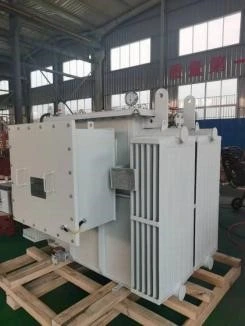# Impact of Distributed Photovoltaic Power Generation Systems On The Operating Characteristics of Distribution Solar Transformers

With Distributed Photovoltaic (DPV) Power Generation Systems, the power supply characteristics change the load characteristics of the traditional distribution network so that the currents have a bi-directional flow.

In order to develop the construction and configuration of distribution solar transformers for active distribution networks, it is necessary to assess the impact of Distributed Photovoltaic (DPV) Power Generation Systems on the operation of distribution solar transformer. The evaluation of the impact of Distributed Photovoltaic (DPV) Power Generation Systems on the operational characteristics of distribution solar transformer has been undertaken.The impact of distributed photovoltaic (DPV) power generation systems on the distributed solar transformer is analysed mechanistically and in order to quantify the impact on the distributed solar transformer, a set of probability-based evaluation indicators is proposed.

A set of probability-based evaluation metrics is proposed to quantify its impact on the distributed solar transformer.

Based on this evaluation index, the variation of voltage, load factor and losses on the LV side of the distribution transformer is investigated under different PV penetration rates.

The impact of Distributed Photovoltaic (DPV) Power Generation Systems on the operating characteristics of the distributed solar transformer is analysed.

The transformer is one of the most important pieces of equipment in the power system as it plays the role of voltage conversion and energy transfer .

With the gradual increase in the penetration of grid-connected photovoltaics, their power supply characteristics have changed the traditional load characteristics.Distributed Photovoltaic (DPV) Power Generation Systems connected to the low-voltage side of the distributed solar transformer (distribution transformer) have an impact on the distribution transformer in two main ways, on the one hand, with the right PV access capacity, the access of PV systems can dissipate On the other hand, when the PV capacity is too high, the distribution solar transformer can be connected to the distribution system.

On the other hand, when the capacity of the PV system is too high or the timing characteristics of the load are different from the timing characteristics of the PV output, it will cause the active power to be backed up, resulting in heavy overloading of the distribution transformer and the voltage on the LV side of the distribution transformer may exceed the upper limit, which in turn reduces the operational reliability of the distribution solar transformer.

At the same time, after the PV system is connected, the pure load characteristics become the characteristics of the PV-load system, which will inevitably have an impact on the operating losses of the distribution solar transformer.

Therefore, it is necessary to establish corresponding evaluation indexes and conduct in-depth research on the situation of PV system access.

We can provide you single phase and three phase pad mounted transformer

#### Dry-type Transformer

Type：Cast resin; Rated Capacity: Up to 25MVA; Rated Voltage: Up to 36KV;

#### Pole Transformer

TypeCSP type Frequency: 50/60Hz; Rated Power: 5~167kva

#### Oil immersed transformer

Frequency: 50/60Hz Rated voltage:10kv, 20kv,30kv Rated Power: 400~2500kva

## Distributed Photovoltaic (DPV) Power Generation Systems access to the distribution solar transformer impact mechanism analysis

### 1.1 Impact on the voltage on the low-voltage side of the distribution substation

In order to facilitate the calculation, the grid connected to the high-voltage side of the distribution substation is simplified to an infinity system, and the load carried by the low-voltage side is shown in Figure 1. Ignore the influence of the fluctuation of the low-voltage side load on the voltage of the high-voltage side of the distribution substation.It can be seen that when the PV is connected to the low-voltage side of the distribution substation, the active power of the system composed of PV and load changes. When the PV penetration rate is small, the active power of the light-load system decreases, the voltage drop decreases and the voltage on the low-voltage side of the distribution substation is raised.

When the PV penetration rate is larger, the active power of the light-load system is backed up, the voltage drop increases and is negative, and the voltage on the low-voltage side of the distribution substation is higher than the rated value, and the upper voltage limit may be crossed.

### Impact on the load factor of the distribution substation

When PV is connected to the low-voltage side of the distribution substation, the active power of the system composed of PV and load changes.

It can be seen that, similar to the analysis of the effect on voltage, when the PV penetration ratio is small, the active power of the light-load system decreases and the distribution substation load factor decreases.

As the PV penetration ratio continues to rise, the active power of the light-load system is fed backwards, the distribution substation load factor rises and heavy overloads may occur.

### Impact on distribution transformer losses

Distribution substation operating losses are mainly divided into two parts: fixed no-load losses P0, and load losses Pk that vary with the load ratio. When PV is connected, the distribution substation operating loss is given by the formulaIt can be seen that when the PV penetration ratio is small, the distribution load ratio decreases and the distribution operating losses decrease.

As the PV penetration ratio continues to increase, the distribution load factor increases and the distribution operating losses increase.

## Probabilistic assessment indicators of the impact of Distributed Photovoltaic (DPV) Power Generation Systems access on distributed solar transformer

In order to quantify the voltage crossing on the LV side of the distribution transformer during steady-state operation of the distribution network containing PV and to analyse the time-series characteristics of the load and PV output, the indicator “probability of voltage crossing on the LV side of the distribution transformer time-series” is defined as the probability that the voltage on the LV side of the distribution transformer is outside the permitted range at each time of the day.In order to grasp the fluctuation range of the voltage on the LV side of the distribution substation at each time of the day, it is necessary to provide reference for the subsequent selection and capacity of the distribution substation. The upper and lower limits of the voltage fluctuation within the confidence interval of the LV side of the distribution substation at each time of the day are defined as the “confidence interval time series of the LV side of the distribution substation” indicator.

### Probabilistic assessment of the impact on the distribution substation load factor

In order to reflect the heavy overload of the distribution substation during steady state operation of the PV distribution network, and to take into account the matching of the load and PV output timing characteristics, and to minimise the heavy overload of the distribution substation in the planning and capacity setting process.The indicator “distribution substation heavy load probability timing” is defined as the probability that the distribution substation load factor is outside the permissible range at all times of the day.

In order to reflect the time series fluctuation range of the substation load rate during the steady state operation of the PV distribution network, to facilitate the viewing of the heavy overload situation of the substation at each time and to provide reference for the subsequent substation selection and capacity setting, the upper and lower limits of the voltage fluctuation within the confidence interval of the substation LV side at each time of the day are defined as the “substation load rate confidence interval time series” indicator.

### Probabilistic assessment index of the impact on distribution losses

When small-capacity PV is connected to the distribution network, some of the local load is absorbed, reducing the transmission power of the distribution substation, which can reduce the operating losses of the distribution substation; when large-capacity PV is connected to the network, because the PV output does not match the timing characteristics of the load, active backfeeding may occur, changing the operating losses of the distribution substation.In order to describe the operating losses of the distribution substation during steady-state operation of the PV-containing distribution network, a “distribution substation confidence daily loss” index is proposed, which is defined as the time series of the maximum fluctuation of the distribution substation operating losses within the confidence interval during a day.

## Analysis of the operating characteristics of distributed solar transformer based on probabilistic evaluation index

In order to demonstrate the influence of Distributed Photovoltaic (DPV) Power Generation Systems on the operating characteristics of the distributed solar transformer, this paper investigates, from the perspective of simulation calculations and based on probabilistic evaluation indexes, the following characteristics of the distributed solar transformer under different boundary conditions of PV access capacity This paper investigates the variation of voltage, load factor and losses on the low voltage side of the distribution solar transformer under different PV access capacity boundary conditions. The simulation method follows the Monte Carlo sampling method.

The PV penetration ratio of the distribution substation is defined as the ratio of the installed PV capacity to the rated capacity of the distribution substation.

Considering the impact of different penetration ratios of PV systems on the distribution transformer, five penetration ratios from 0% to 100% are set, an oil-immersed distribution solar transformer S13 with a capacity of 400 k VA is selected, the rated load is set to 350 kVA, and the expected value/standard deviation of the voltage on the high-voltage side of the distribution transformer is set to 1.04(p.u.)/0.01. 0.01.As the PV penetration rate increases, the greater the PV output, the greater the effect on the voltage lift. This is reflected in the upper and lower limits of the voltage confidence interval on the low-voltage side of the distribution substation during daylight hours as the PV penetration rate continues to increase.

(1) After the PV is connected to the grid, the distribution substation load factor decreases to a certain extent, and the greater the PV penetration rate, the more obvious the decrease in load factor at that moment.

(2) As the PV penetration rate increases, the distribution substation load factor shows an overall trend of decrease. And at 13, there was a phenomenon that the fluctuation range of the distribution load rate for a PV penetration rate of 75% was lower than that of the distribution load rate for a penetration rate of 100%. This is due to the fact that at 100% PV penetration, the load factor increases due to the power backfeed from the distribution substation.

As the PV penetration rate increases, the PV power output increases, the PV’s ability to consume local load is enhanced, the distribution substation load factor is reduced, and its confidence daily loss is also reduced.

## ConclusionThe impact of Distributed Photovoltaic (DPV) Power Generation Systems on the operating characteristics of distributed solar transformer has been studied from a probabilistic perspective, using PV as a typical representative of various distributed power sources.

Firstly, the effect of access to Distributed Photovoltaic (DPV) Power Generation Systems on the operating characteristics of distribution solar transformer is analysed mechanistically.

Then, a set of probabilistic evaluation indicators is proposed to quantitatively evaluate the impact of distributed photovoltaic (DPV) power generation systems on the operating characteristics of distributed solar transformer under different boundary conditions of PV penetration, which will provide a reference for the distribution network operation planning. The study will provide a reference for the operational planning of the distribution network.

### 10 kv Oil Filled Transformer

Fault Diagnosis and Analysis of 10 kv Oil Filled Transformer 10 kv Oil filled transformer